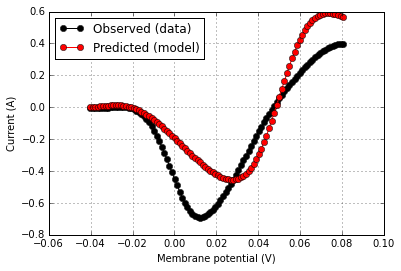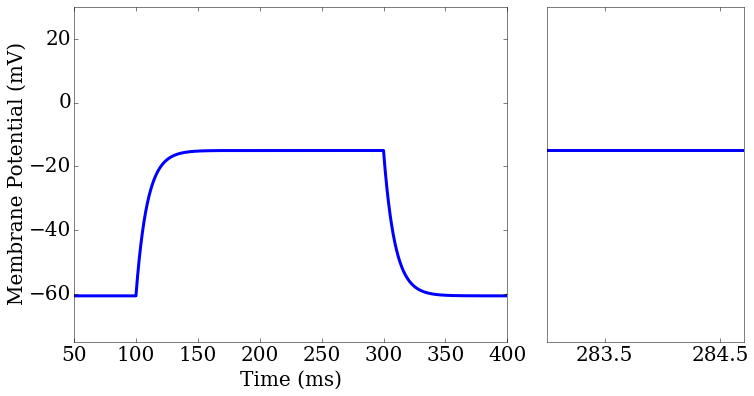Welcome to NeuronUnit’s documentation!NeuronUnit Logo

# Concept:¶

NeuronUnit uses the SciUnit-framework to test models of ion channels, neurons, and neuronal networks.

# Documentation:¶

(See SciUnit documentation here first) - Chapter 1 - Chapter 2 - Chapter 3

# Examples:¶

## (Example 1) Validating an ion channel model’s IV curve against data from a published experiment¶

```from channelworm.ion_channel.models import GraphData
from neuronunit.tests.channel import IVCurvePeakTest
from neuronunit.models.channel import ChannelModel

# Instantiate the model
channel_model_name = 'EGL-19.channel' # Name of a NeuroML channel model
channel_id = 'ca_boyle'
channel_file_path = os.path.join('path', 'to', 'models', '%s.nml' % channel_model_name)
model = ChannelModel(channel_file_path, channel_index=0, name=channel_model_name)

# Get the experiment data from ChannelWorm and instantiate the test
doi = '10.1083/jcb.200203055'
fig = '2B'
sample_data = GraphData.objects.get(graph__experiment__reference__doi=doi,
patch_capacitance = pq.Quantity(1e-13,'F') # Assume recorded patch had this capacitance;
# an arbitrary scaling factor.
observation = {'v':voltage,
'i':current}
test = IVCurvePeakTest(observation)

# Judge the model output against the experimental data
score = test.judge(model)
rd = score.related_data
score.plot(rd['v'],rd['i_obs'],color='k',label='Observed (data)')
score.plot(rd['v'],rd['i_pred'],same_fig=True,color='r',label='Predicted (model)')
```png

```score.summarize()
""" OUTPUT:
Model EGL-19.channel (ChannelModel) achieved score Fail on test 'IV Curve Test (IVCurvePeakTest)'. ===
"""
score.describe()
""" OUTPUT:
The score was computed according to 'The sum-squared difference in the observed and predicted current values over the range of the tested holding potentials.' with raw value 3.151 pA^2
"""
```

## (Example 2) Testing the membrane potential and action potential widths of several spiking neuron models against experimental data found at http://neuroelectro.org.¶

```import sciunit
from neuronunit import neuroelectro,tests,capabilities
import neuronunit.neuroconstruct.models as nc_models
from pythonnC.utils.putils import OSB_MODELS

# We will test cerebellar granule cell models.
brain_area = 'cerebellum'
neuron_type = 'cerebellar_granule_cell'
path = os.path.join(OSB_MODELS,brain_area,neuron_type)
neurolex_id = 'nifext_128' # Cerebellar Granule Cell

# Specify reference data for a test of resting potential for a granule cell.
reference_data = neuroelectro.NeuroElectroSummary(
neuron = {'nlex_id':neurolex_id}, # Neuron type.
ephysprop = {'name':'Resting Membrane Potential'}) # Electrophysiological property name.
# Get and verify summary data for the combination above from neuroelectro.org.
reference_data.get_values()
vm_test = tests.RestingPotentialTest(
observation = {'mean':reference_data.mean,
'sd':reference_data.std},
name = 'Resting Potential')

# Specify reference data for a test of action potential width.
reference_data = neuroelectro.NeuroElectroSummary(
neuron = {'nlex_id':neurolex_id}, # Neuron type.
ephysprop = {'name':'Spike Half-Width'}) # Electrophysiological property name.
# Get and verify summary data for the combination above from neuroelectro.org.
reference_data.get_values()
spikewidth_test = tests.InjectedCurrentAPWidthTest(
observation = {'mean':reference_data.mean,
'sd':reference_data.std},
name = 'Spike Width',
params={'injected_square_current':{'amplitude':5.3*pq.pA,
'delay':50.0*pq.ms,
'duration':500.0*pq.ms}})
# 5.3 pA of injected current in a 500 ms square pulse.

# Create a test suite from these two tests.
suite = sciunit.TestSuite('Neuron Tests',(spikewidth_test,vm_test))

models = []
for model_name in model_names # Iterate through a list of models downloaded from http://opensourcebrain.org
model_info = (brain_area,neuron_type,model_name)
model = nc_models.OSBModel(*model_info)
models.append(model) # Add to the list of models to be tested.

score_matrix = suite.judge(models,stop_on_error=True)
score_matrix.view()
```

## Score Matrix for Test Suite ‘Neuron Tests’¶

Model Spike Width Resting Potential
(InjectedCu rrentSpikeWidthTest) (R estingPotentialTest)
cereb_grc_mc (OSBModel) Z = nan Z = 4.92
GranuleCell (OSBModel) Z = -3.56 Z = 0.88
```import matplotlib.pyplot as plt
ax1 = plt.subplot2grid((1,3), (0,0), colspan=2)
ax2 = plt.subplot2grid((1,3), (0,2), colspan=1)
rd = score.related_data
score_matrix[0,0].plot(rd['t'],rd['v_pred'],ax=ax1)
score_matrix[0,1].plot(rd['t'],rd['v_pred'],ax=ax2)
ax2.set_xlim(283,284.7)
```# Tutorial:¶

NeuronUnit is based on SciUnit, a discipline-agnostic framework for data-driven unit testing of scientific models. Any test script will do the following things in sequence. Most of these will be abstracted away in SciUnit or NeuronUnit modules that make things easier:

1. Instantiate a model(s) from a model class, with parameters of interest to build a specific model.
2. Instantiate a test(s) from a test class, with parameters of interest to build a specific test.
3. Check that the model has the capabilities required to take the test.
4. Make the model take the test.
5. Generate a score from that test run.
6. Bind the score to the specific model/test combination and any related data from test execution.
7. Visualize the score (i.e. print or display the result of the test).

Here, we will break down how this is accomplished in NeuronUnit. Although NeuronUnit contains several model and test classes that make it easy to work with standards in neuron modeling and electrophysiology data reporting, here we will use toy model and test classes constructed on-the-fly so the process of model and test construction is fully transparent.

Here is a toy model class:

```class ToyNeuronModel(sciunit.Model,
neuronunit.capabilities.ProducesMembranePotential):
"""A toy neuron model that is always at its resting potential"""
def __init__(self, v_rest, name=None):
self.v_rest = v_rest
sciunit.Model.__init__(self, name=name)

def get_membrane_potential(self):
array = np.ones(10000) * self.v_rest
dt = 1*ms # Time per sample in milliseconds.
vm = AnalogSignal(array,units=mV,sampling_rate=1.0/dt)
return vm
```

The `ToyNeuronModel` class inherits from `sciunit.Model` (as do all NeuronUnit models), and also from `ProducesMembranePotential`, which is a subclass of `sciunit.Capability`. Inheriting from a SciUnit `Capability` is a how a SciUnit `Model` lets tests know what it can and cannot do. It tells tests that the model will be implementing any method stubbed out in the definition of that `Capability`.

Let’s see what the `neuronunit.capabilities.ProducesMembranePotential` capability looks like:

```class ProducesMembranePotential(Capability):
"""Indicates that the model produces a somatic membrane potential."""

def get_membrane_potential(self):
"""Must return a neo.core.AnalogSignal."""
raise NotImplementedError()

def get_median_vm(self):
vm = self.get_membrane_potential()
return np.median(vm)
```

`ProducesMembranePotential` has two methods. The first, `get_membrane_potential` is unimplemented by design. Since there is no way to know how each model will generate and return a membrane potential time series, the `get_membrane_potential` method in this capability is left unimplemented, while the docstring describes what the model must implement in order to satisfy that capability. In the `ToyNeuronModel` above, we see that the model implements it by simply creating a long array of resting potential values, and returning it as a `neo.core.AnalogSignal` object.

The second, `get_median_vm` is implemented, which means there is no need for the model to implement it again. For its implementation to work, however, the implementation of `get_membrane_potential` must be complete. Pre-implemented capability methods such as these allow the developer to focus on implementing only a few core interactions with the model, and then getting a lot of extra functionality for free. In the example above, once we know that the membrane potential is being returned as a `neo.core.AnalogSignal`, we can simply take the median using numpy. We know that the membrane potential isn’t being returned as a list or a tuple or some other object on which numpy’s median function won’t necessarily work.

Let’s construct a single instance of this model, by choosing a value for the single membrane potential argument. This toy model will now have a 60 mV membrane potential at all times:

```from quantities import mV
my_neuron_model = ToyNeuronModel(-60.0*mV, name='my_neuron_model')
```

Now we can then construct a simple test to use on this model or any other test that expresses the appropriate capabilities:

```class ToyAveragePotentialTest(sciunit.Test):
"""Tests the average membrane potential of a neuron."""

def __init__(self,
observation={'mean':None,'sd':None},
name="Average potential test"):
"""Takes the mean and standard deviation of reference membrane potentials."""

sciunit.Test.__init__(self,observation,name) # Call the base constructor.
self.required_capabilities += (neuronunit.capabilities.ProducesMembranePotential,)
# This test will require a model to express the above capabilities

description = "A test of the average membrane potential of a cell."
score_type = sciunit.scores.ZScore # The test will return this kind of score.

def validate_observation(self, observation):
"""An optional method that makes sure an observation to be used as
reference data has the right form"""
try:
assert type(observation['mean']) is quantities.Quantity # From the 'quantities' package
assert type(observation['sd']) is quantities.Quantity
except Exception as e:
raise sciunit.ObservationError(("Observation must be of the form "
"{'mean':float*mV,'sd':float*mV}"))

def generate_prediction(self, model):
"""Implementation of sciunit.Test.generate_prediction."""
vm = model.get_median_vm() # If the model has the capability 'ProducesMembranePotential',
# then it implements this method
prediction = {'mean':vm}
return prediction

def compute_score(self, observation, prediction):
"""Implementation of sciunit.Test.score_prediction."""
score = sciunit.comparators.zscore(observation,prediction)  # Computes a decimal Z score.
score = sciunit.scores.ZScore(score) # Wraps it in a sciunit.Score type.
score.related_data['mean_vm'] = prediction['mean'] # Binds some related data about the test run.
return score
```

The test constructor takes an observation to parameterize the test, e.g.:

```from quantities import mV
my_observation = {'mean':-60.0*mV,
'sd':3.5*mV}
my_average_potential_test = ToyAveragePotentialTest(my_observation, name='my_average_potential_test')
```

A few things happen upon test instantiation, including validation of the observation. Since the observation has the correct format (for this test class), `ToyAveragePotentialTest.validate_observation` will complete successfully and the test will be ready for use.

```score = my_average_potential_test.judge(my_neuron_model)
```
The `sciunit.Test.judge` method does several things.
– First, it checks to makes sure that my_neuron_model expresses the capabilities required to take the test. It doesn’t check to see if they are implemented correctly (how could it know?) but it does check to make sure the model at least claims (through inheritance) to express these capabilities. The required capabilities are none other than those in the test’s `required_capabilities` attribute. Since `ProducesMembranePotential` is the only required capability, and the `ToyNeuronModel` class inherits from this capability class, that check passes.
– Second, it calls the test’s `generate_prediction` method, which uses the model’s capabilities to make the model return some quantity of interest, in this case the median membrane potential.
– Third, it calls the test’s `compute_score` method, which compares the observation the test was instantiated with against the prediction returned in the previous step. This comparison of quantities is cast into a `score` (in this case, a Z Score), bounds to some model output of interest (in this case, the model’s mean membrane potential), and that `score` object is returned.
– Fourth, the score returned is checked to make sure it is of the type promised in the class definition, i.e. that a Z Score is returned if a Z Score is listed in the test’s `score_type` attribute.
– Fifth, the score is bound to the test that returned it, the model that took the test, and the prediction and observation that were used to compute it.

If all these checks pass (and there are no runtime errors) then we will get back a `score` object. Usually this will be the kind of score we can use to evaluate model/data agreement. If one of the capabilities required by the test is not expressed by the model, `judge` returns a special `NAScore` score type, which can be thought of as a blank. It’s not an error – it just means that the model, as written, is not capable of taking that test. If there are runtime errors, they will be raised during test execution; however if the optional `stop_on_error` keyword argument is set to `False` when we call `judge`, then it we will return a special `ErrorScore` score type, encoding the error that was raised. This is useful when batch testing many model and test combinations, so that the whole script doesn’t get halted. One can always check the scores at the end and then fix and re-run the subset of model/test combinations that returned an `ErrorScore`.

For any score, we can summarize it like so:

```score.summarize()
''' OUTPUT:
=== Model my_neuron_model (ToyAveragePotentialModel) achieved score 1.0 on test my_average_potential_test (ToyAveragePotentialTest)'. ===
'''
```

```score.describe()